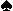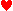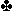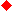# Duplicate Bridge Scoring

The basic formula is:

 50 points for making a contract + 10 extra for notrump, + 20 / trick in a minor suit, + 30 / trick in a major suit or notrump.
 EXAMPLES: 2= 50 + 2 × 30 = 110 3= 50 + 3 × 30 = 140 1NT = 50 + 10 + 30 = 90 2NT = 50 + 10 + 2 × 30 = 120 2= 50 + 2 × 20 = 90

Contract Independence.     As long as you make your contract, your score depends only on the number of tricks you made, and not on the contract you bid. For example, 1making 3 and 3making 3 are both worth 140 points. Therefore, it is in your interests to bid a low-level contract, and it is in your opponents' interests to force you into a high-level contract.

Game.     Certain high-level contracts are worth bonus points. For example:

 Bidding and making a game contract is worth 250 bonus points.

The game contracts are as follows:

 Game Contracts 4 of a major (4or 4) 5 of a minor (5or 5) 3 notrumps

Note that you must bid a game contract (or higher) to get the 250 point bonus (e.g. 1making 4 is only worth 170). This is an important exception to the principle of "contract independence".

 EXAMPLES: 4making 4 = 50 + 4 × 30 + 250 = 420 5making 5 = 50 + 4 × 30 + 250 = 400 3NT making 3 = 50 + 10 + 3 × 30 + 250 = 400

Note that 3NT making 3 is worth the same as 5 of a minor making 5. However, 3NT only requires you to take 9 tricks, while you must take 11 tricks to make 5or 5. For this reason, when you have a minor-suit fit, it is often better to be in 3NT than in minor-suit game.

Slam.     A slam is a contract at the 6-level or 7-level. Slam contracts are worth additional bonus points (beyond the game bonus):

 A small slam is a contract at the 6-level. Bidding and making a small slam is worth 500 bonus points. A grand slam is a contract at the 7-level. Bidding and making a grand slam is worth 1000 bonus points.

For example, 7making 7 is worth 50 + 7 × 30 + 250 + 1000 = 1510 points.

NOTE:    When calculating such values, it is often easier to recall that 4making 4 is worth 420. For example:

 7making 7 = 420 + 3 × 30 + 1000 = 1510

Going Down.     When you fail to make your contract, it is called going down, and the extra tricks that you failed to take are called undertricks. When you go down in a contract, your opponents receive points:

 –50 point penalty for each undertrick.

Doubles.     If your opponents think you will go down, they can double you to increase the penalty. Penalties for going down in a doubled contract are quite severe:

 Penalties for Going Down Doubled –100 for going down 1 doubled. –300 for going down 2 doubled. –500 for going down 3 doubled. –800 for going down 4 doubled. –300 more for each additional undertrick.

However, if your opponents double and you make the contract, then you receive extra points:

 Points for Making a Doubled Contract 2 × (points for the contract as though it were a partscore), + 100 points for each overtrick, + 250 point game bonus for 2, 2, 3, 3, or 2NT, + normal bonus for slams (if applicable).

Note that 2 of a major doubled is game, as is 3 of a minor or 2NT. When you bid such a contract and are doubled, you have been doubled into game, and you receive the full game bonus.

 EXAMPLES: 1.    3is worth 140, so 3doubled making 4 is 2 × 140 + 250 + 100 = 630! 2.    3NT is worth 50 + 10 + 3 × 30 = 150 points as a partscore, so 3NT doubled is 2 × 150 + 250 = 550!

Note that the more silly the opponents' double, the more points you get. For example, 2doubled making 4 is worth 670, but 4doubled making 4 is only worth 590. Therefore, once the opponents double you into a game contract, there is no reason to bid further unless you think you can make slam.

Redoubles.     If your opponents double a contract you think you will make, you can redouble for even more points:

 Points for Making a Redoubled Contract 4 × (points for the contract as though it were a partscore), – 50 + 200 points for each overtrick, + 250 point game bonus for 1, 1, 2, 2, or 1NT, + normal bonus for slams (if applicable).

However, it is disastrous to go down in a redoubled contract:

 Going down in a redoubled contract is worth twice the penalty of going down doubled.

Vulnerability.      About half of the time, you will be vulnerable. This has three important consequences:

1.    You get more bonus points for making game or slam:

 A vulnerable game is worth a 450 point bonus. A vulnerable small slam is worth a 750 point bonus. A vulnerable grand slam is worth a 1500 point bonus.

2.    You lose more points for going down:

 –100 point penalty for each vulnerable undertrick.

3.    All doubles are more costly:

 Penalties for Going Down Doubled Vulnerable –200 for going down 1 doubled vulnerable. –500 for going down 2 doubled vulnerable. –800 for going down 3 doubled vulnerable. –1100 for going down 4 doubled vulnerable. –300 more for each additional undertrick.

On the other hand:

 +200 points per overtrick when doubled vulnerable. +400 points per overtrick when redoubled vulnerable. +450 point bonus for being doubled or redoubled into game.